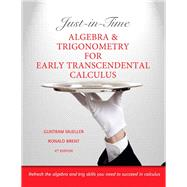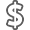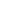# Just-in-Time Algebra and Trigonometry for Early Transcendentals Calculus

• ISBN13:

• ISBN10:

## 0321671031

• Edition: 4th
• Format: Paperback
• Publisher: Pearson

Note: Supplemental materials are not guaranteed with Rental or Used book purchases.

Purchase Benefits

•Free Shipping On Orders Over \$35!
Your order must be \$35 or more to qualify for free economy shipping. Bulk sales, PO's, Marketplace items, eBooks and apparel do not qualify for this offer.
•Get Rewarded for Ordering Your Textbooks! Enroll Now
•We Buy This Book Back!
In-Store Credit: \$5.25
Check/Direct Deposit: \$5.00
PayPal: \$5.00
List Price: \$33.32 Save up to \$16.66
• Rent Book \$16.66Free Shipping

TERM
PRICE
DUE
CURRENTLY AVAILABLE, USUALLY SHIPS IN 24-48 HOURS
*This item is part of an exclusive publisher rental program and requires an additional convenience fee. This fee will be reflected in the shopping cart.

### Supplemental Materials

What is included with this book?

• The New copy of this book will include any supplemental materials advertised. Please check the title of the book to determine if it should include any access cards, study guides, lab manuals, CDs, etc.
• The Used and Rental copies of this book are not guaranteed to include any supplemental materials. Typically, only the book itself is included. This is true even if the title states it includes any access cards, study guides, lab manuals, CDs, etc.

### Summary

Are you ready to ace calculus at the college level? With this book, you will be! Professors often say "Students don't fail the calculus, they fail the algebra." In other words, even if you understand calculus, your algebra and trigonometry skills can hold you back. Here's a quick quiz-do you remember how to: Factor trinomials? Solve equations containing exponents and logs? Work with inverse trig functions? If not, that's where this book comes in handy! Just-in-Timeis designed to bolster the algebra and trigonometry skills you'll need while you study calculus. As you make your way through the course, Just-in-Timeis with you every step of the way, showing you the exact algebra or trigonometry topics that you'll need and pointing out potential problem spots. The easy-to-use Table of Contents features the calculus subject listed directly across from the algebra/trigonometry skills needed to master that topic. Use this book as your study companion and put your anxiety to rest!

### Author Biography

Guntram B. Mueller received his PhD in Mathematics from the University of Notre Dame. He has taught calculus many times and is very familiar with the typical strengths and weaknesses in the backgrounds of his students. That experience is what guided him, in cooperation with Dr. Ron Brent, in writing the Just-in-Time series. His advice? Buy the book! It's got just what you need, just in time.

Ronald I. Brent is a Professor of Mathematics at University of Massachusetts, Lowell, where he has taught since 1987. He earned his PhD in Mathematics from Rensselaer Polytechnic Institute. Dr. Brent is the author of many publications, including three Just-In-Time mathematics texts. His main advice to his calculus students is: “Work on your homework as if your life in this course depends upon it. Because it does!

Calculus Topic: Review of Basics

1. Numbers and Their Disguises

Multiplying and dividing fractions, adding and subtracting fractions, parentheses, exponents, roots, percent, scientific notation, calculators, rounding, intervals

Calculus Topic: Circles, Parabolas, etc.

2. Completing the Square

Completing the square in one and two variables

Calculus Topic: Equations

3. Solving Equations

Equations of degree 1 and 2, solving other types of equations, rational equations, the zero-factor property

Calculus Topic: Algebraic Functions and Graphs

4. Functions and Their Graphs

Introduction, equations of lines, power functions, shifting graphs, intersection of curves

Calculus Topic: Transcendental Functions

5. Cyclic Phenomena: The Six Basic Trigonometric Functions

Angles, definitions of the six trigonometric functions, basic identities, special angles, sum formulas, graphs of complex trigonometric functions

6. Exponential Functions

The family of exponentials, the function ex

7. Composition and Inverse Functions

Composite functions, the idea of inverses, finding an inverse of f given by a graph, finding the inverse of f given by an expression

8. Logarithmic Functions

Definition of logarithms, logs as inverses of exponential functions, laws of logarithms, the natural logarithm

9. Inverse Trigonometric Functions

The definition of arcsin x, the functions arctan x and arcsec x, inverse trigonometric identities

Calculus Topic: Limits

10. Changing the Form of a Function

Factoring, canceling, long division, rationalizing, extracting a factor from under a root

Calculus Topic: Derivatives

11. Simplifying Algebraic Expressions

Working with difference quotients and rational functions, canceling common factors, rationalizing expressions

Calculus Topic: The Chain Rule

12. Decomposition of Functions

Inner, outer, and outermost functions, decomposing composite functions

Calculus Topic: Implicit Differentiation

13. Equations of Degree 1 Revisited

Solving linear equations involving derivatives

Calculus Topic: Related Rates, Applied Max-Min Problems

14. Word Problems, Algebraic and Transcendental

Applied Max-Min Problems, Algebraic word problems, the geometry of rectangles, circles and spheres, trigonometric word problems, right angle triangles, the law of sines and the law of cosines, exponential growth and decay

Calculus Topic: Integrating Trigonometric Functions

15. Trigonometric Identities

Rewriting trigonometric expressions using identities.

Appendices

A. The Binomial Theorem

B. Derivation of The Quadratic Formula